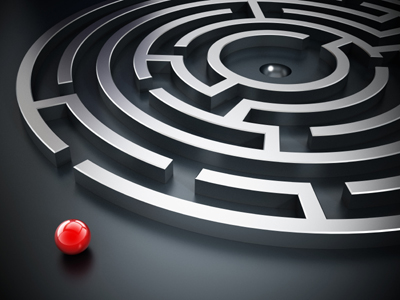Test your knowledge of perimeter in this quiz.

# Perimeter (F)

Perimeter and area, two properties of two-dimensional shapes that are easily mixed up. If you have already tried the Area quiz you should remember the difference – area is the amount of space a shape takes up, whilst perimeter is the distance around the outside of it. Try this GCSE Maths quiz and see if you make the distance!

When working out the perimeter of a shape with straight edges, it is a good idea to mark in the length of each edge. Sometimes, particularly with complex shapes, not all the lengths are given and you have to work them out. Once you have them all in place, start at one corner and imagine walking all the way round the shape and back to the start. Total up the distance you have travelled, and that is your perimeter! Don’t forget to include units – as it is a length, this will usually be metres or cm.

The perimeter of a circle has a special name and a formula to go with it, so it’s important that you remember this. If you are asked for the perimeter of a shape that includes part of a circle, for example a quarter circle, don’t forget to add in the straight edges!

Question 1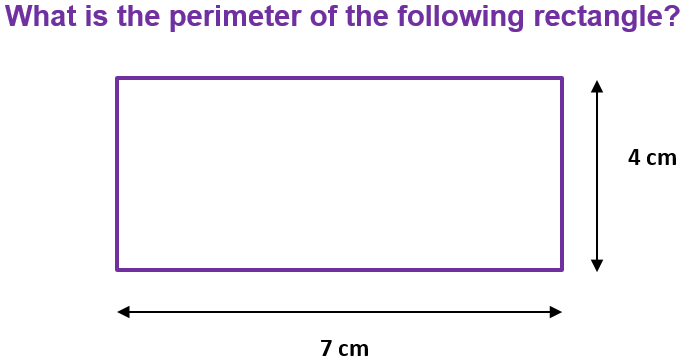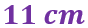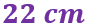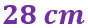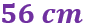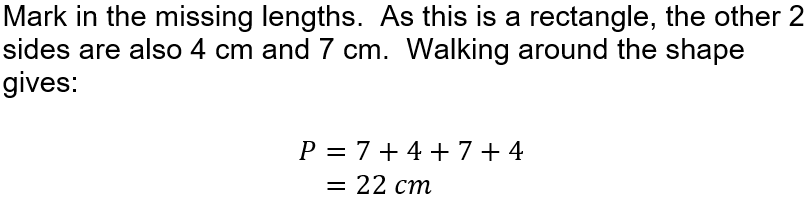Question 2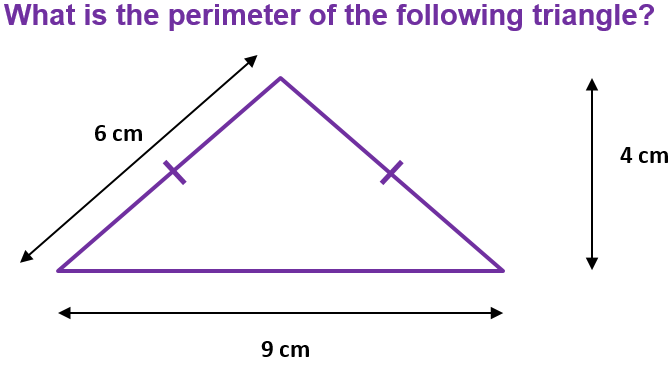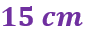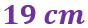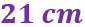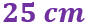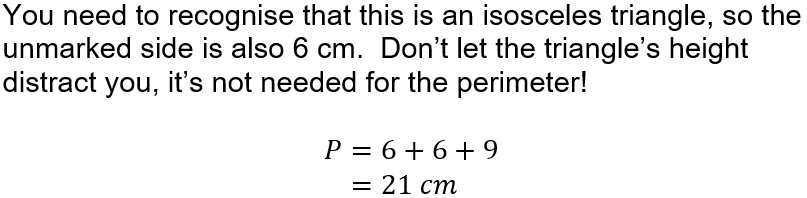Question 3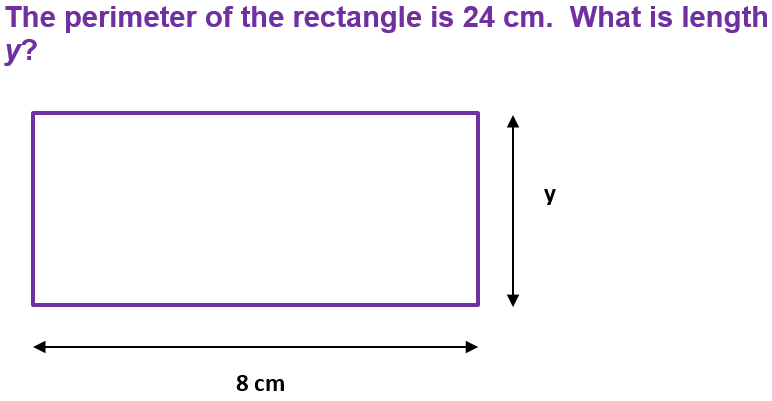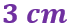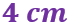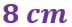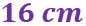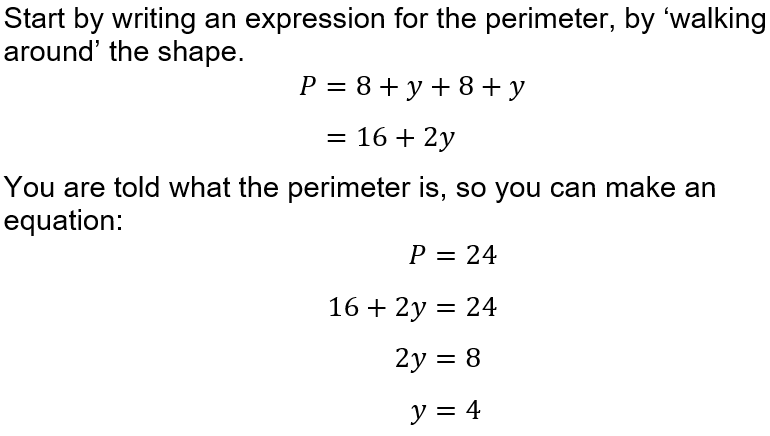Question 4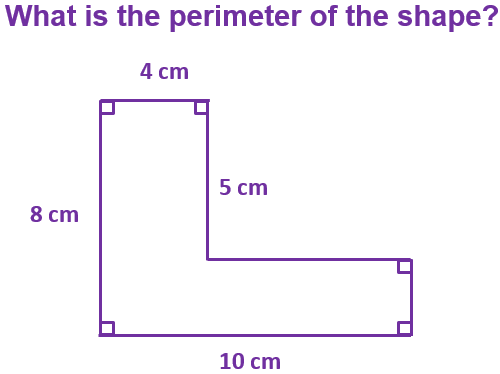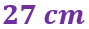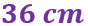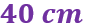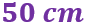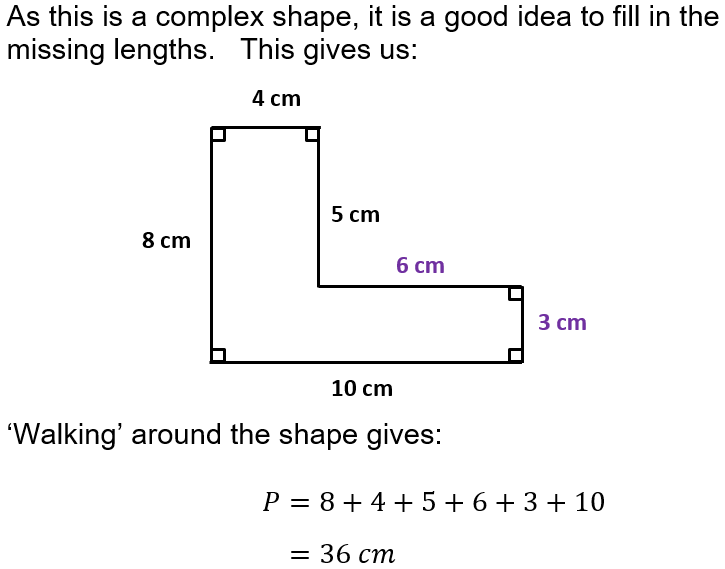Question 5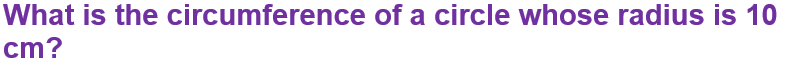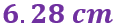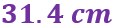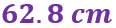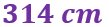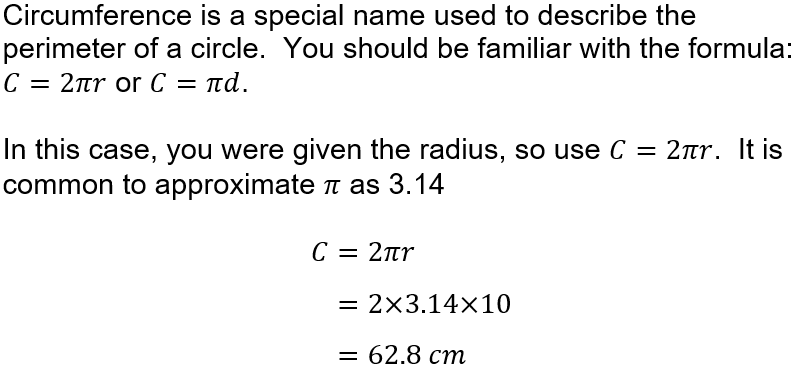Question 6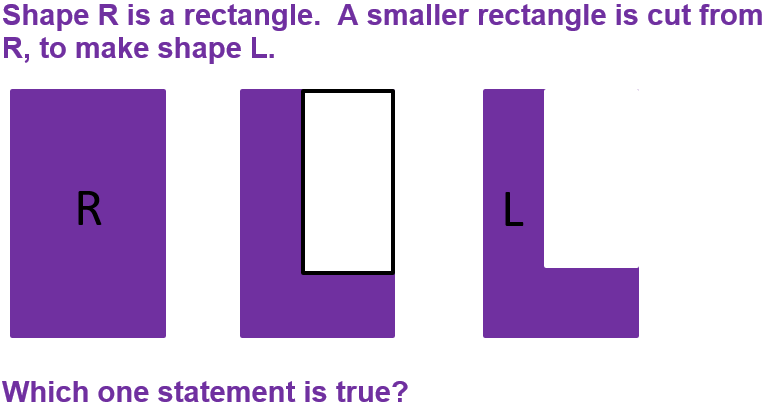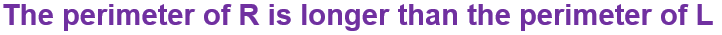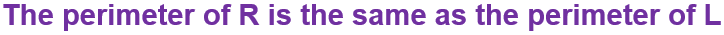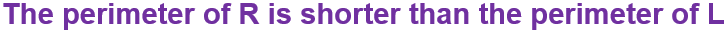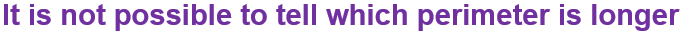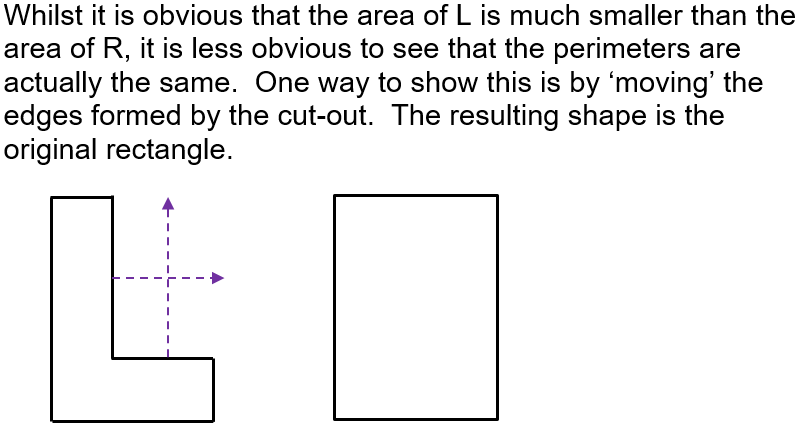Question 7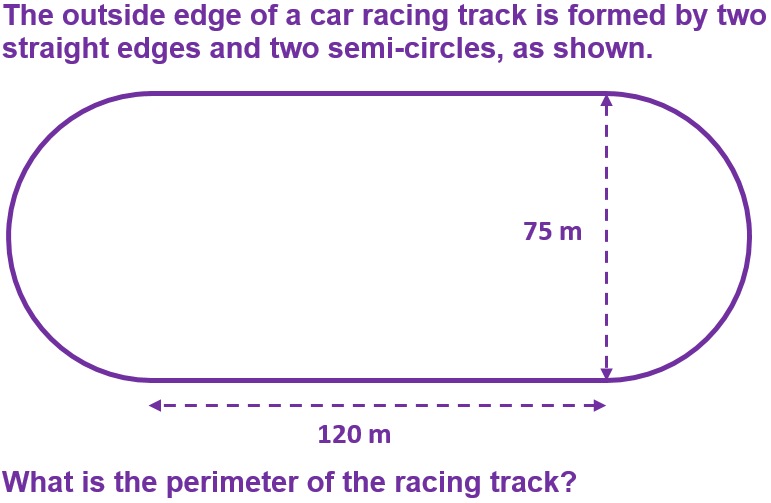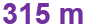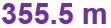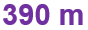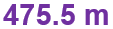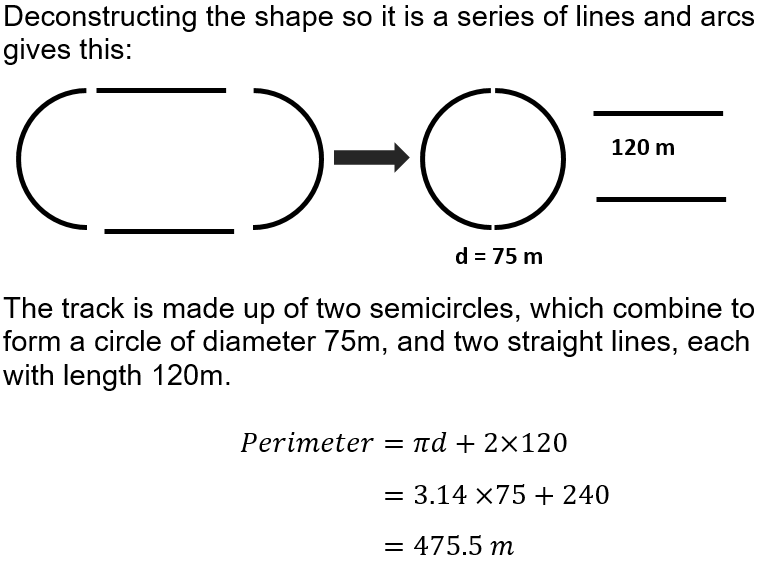Question 8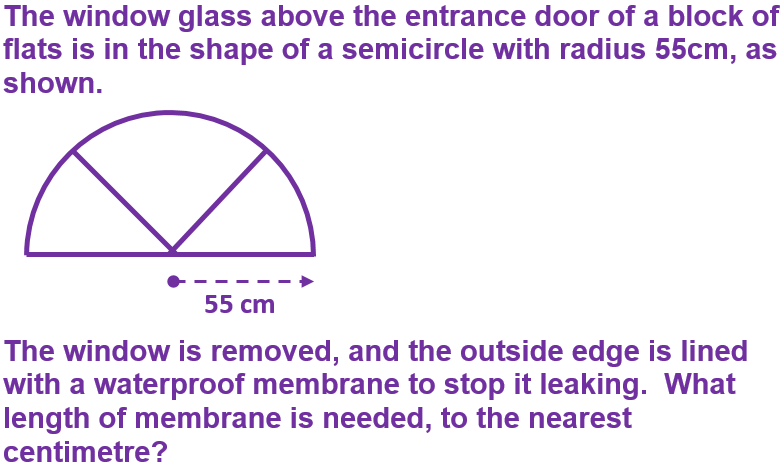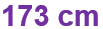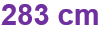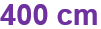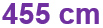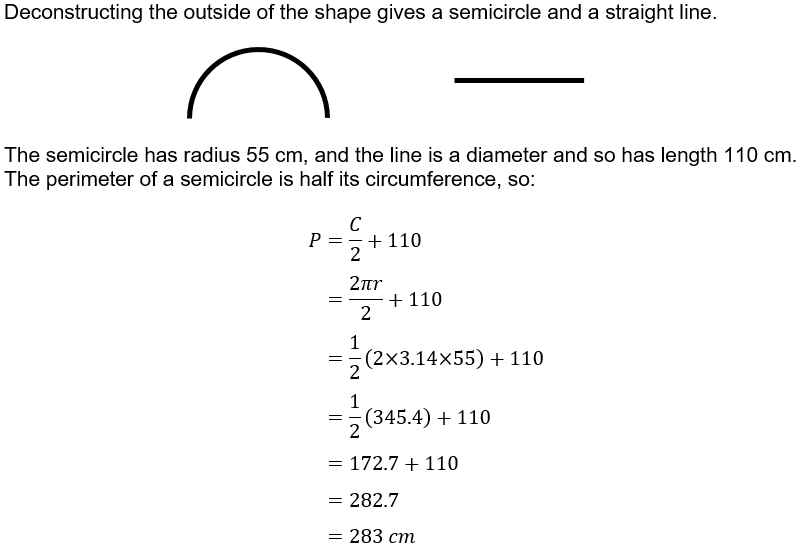Question 9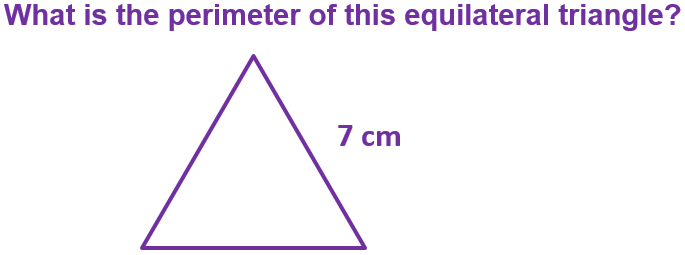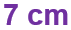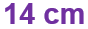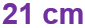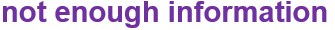Question 10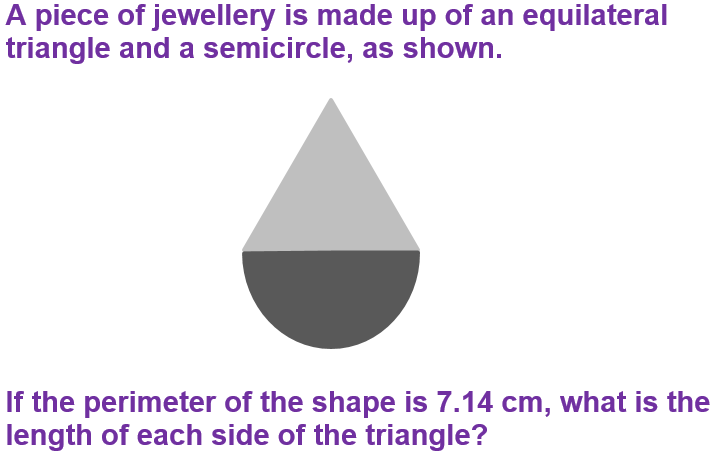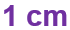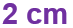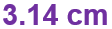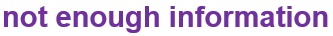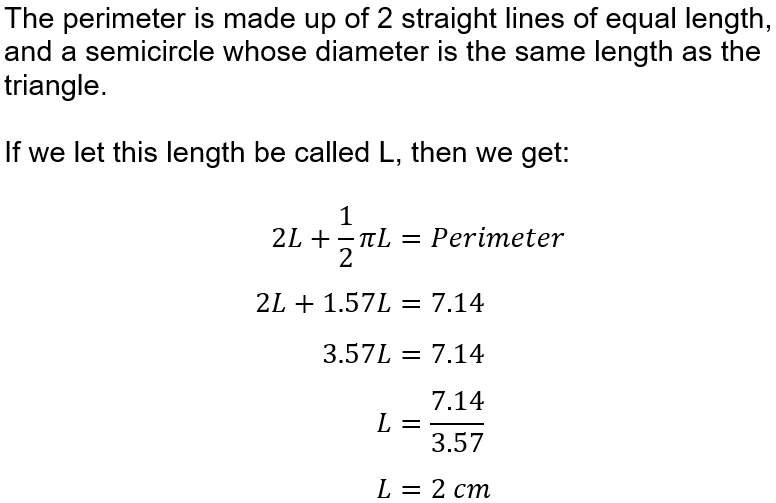Author:  Sally Thompson